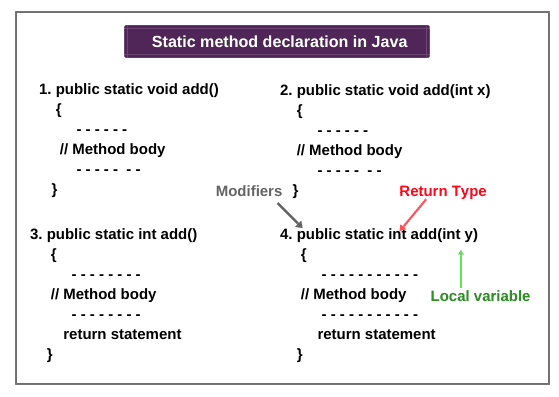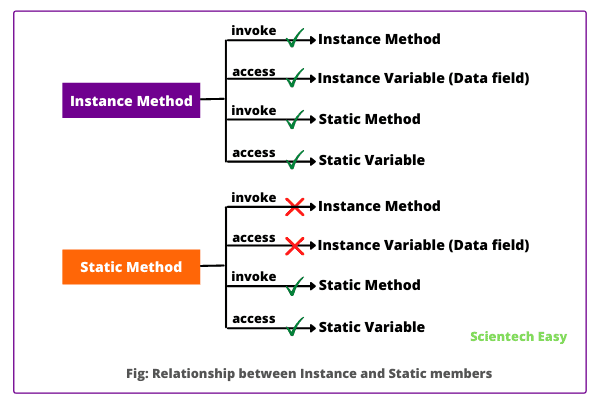# Static Method in Java | Example Program

When a method is declared with the keyword ‘static’, it is called static method in java.

Like a static variable, static method is also tied to the class, not to an object of class. Therefore, it can be called and executed without creating objects of the class.

Static method can be called or accessed directly using class name. The syntax to call a static method in Java is as follows:

```className.methodName(); // Here, className is the name of a class.
For example:
Student.add(); // Student is the name of class and add is a method.```

A static method is also known as class method in java because it belongs to a class rather than an individual instance of a class.

## Declaration of Static method in Java

The general syntax to declare the static method ( or class method) in java is as follows:

```Access_modifier static void methodName()
{ // block start
. . . . . . . . . .
// Method body.
. . . . . . . . . .
} // block end.```

The different forms of declaration of a static method with or without return type are shown in the below figure.## Features of Static method (Class method)

There are several important features of a static method in java that must keep in mind. They are as follows:

1. A static method in a class can directly access other static members of the class. We do not need to create an object of class for accessing other static members. It can be called directly within the same class and outside the class using the class name.

2. It cannot access instance (i.e. non-static) members of a class. The relationship between instance and static members are summarized in the below figure:From the figure, it is clear that:

• An instance method can call an instance or static method. It can also access instance or static data variable.
• A static method can call a static method only. It can only access a static data variable inside the static method.
• A static method cannot invoke an instance method or access an instance variable.

3. We cannot declare a static method and instance method with the same signature in the same class hierarchy.

4. When we create a static method in the class, only one copy of the method is created in the memory and shared by all objects of the class. Whether you create 100 objects or 1 object.

5. A static method in java is also loaded into the memory before the object creation.

6. The static method is always bound with compile time.

7. It cannot refer to this or super in any way.

8. Static methods can be overloaded in Java but cannot be overridden because they are bound with class, not instance.

9.  Static (variable, method, and inner class) are stored in Permanent generation memory (class memory).

## Why Instance variable is not available to Static method?

When we declare a static method in Java, JVM first executes the static method, and then it creates objects of the class. Since objects are not available at the time of calling the static method.

Therefore, instance variables are also not available to a static method. Due to which a static method cannot access an instance variable in the class.

Let’s take an example program to test whether a static method can access an instance variable and static variable or not.

In this program, we will try to read and display instance variable ‘y’ and static variable ‘x’ of class StaticTest in an instance method ‘display()’ and static method ‘show()’.

Program source code 1:

```package staticMethod;
public class StaticTest
{
// Instance Area.
static int x = 20; // static variable
int y = 30; // instance variable
// Declare an instance method.
void display()
{
// Instance area. So we can directly call instance variable without using object reference variable.
System.out.println(x); // Since we can access static member within instance area. Therefore, we can call the static variable directly.
System.out.println(y);
}
// Declare a static method.
static void show()
{
// Static Area. So we can call S.V. directly inside the S.M.
System.out.println(x);
// System.out.println(y); // compile time error because instance variable cannot access inside S.M.
}
public static void main(String[] args)
{
// Create the object of the class.
StaticTest st = new StaticTest();
// Call instance method using reference variable st.
st.display();

// Call static method.
show();
}
}```
```Output:
20
30
20```

The static method can be accessed by nullable reference as:

```StaticMethod s1 = null;
s1.show();```

Let’s take an example program based on it.

Program source code 2:

```package staticMethod;
public class StaticMethod
{
static int a = 10;
void display()
{
System.out.println("This is an instance method");
}
static void show()
{
System.out.println("This is a Static method");
}
public static void main(String[] args)
{
StaticMethod sm = new StaticMethod();
sm.display();
StaticMethod s = null;
s.show();
int c = s.a;
System.out.println(c);
}
}```
```Output:
This is an Instance method
This is a Static method
10```

## How to change value of static variable inside static method?

Static method can access a static variable and can also change its value.

Let’s write a program where we will change the value of static variable inside static method. Look at the following source code.

Program source code 3:

```package staticMethod;
public class ValueChange
{
static int a = 10;
static int change()
{
int a = 20;
return a;
}
public static void main(String[] args)
{
// Call static method using the class name. Since it will return an integer value. So we will store it by using a changeValue variable.
int changeValue = ValueChange.change();
System.out.println(changeValue);
}
}```
```Output:
20```

Let’s take another program where we will calculate the square and cube of a given number by using static method.

Program source code 4:

```package staticMethod;
public class SquareAndCube
{
static int x = 15;
static int y = 20;
static int square(int x)
{ // Here, x is a local variable.
int a = x * x;
return a;
}
static int cube(int y){ // Here, y is a local variable.
int b = y*y*y;
return b;
}
public static void main(String[] args)
{
int sq = square(5);
int CB = cube(10);
System.out.println(sq);
System.out.println(cb);
}
}```
```Output:
25
1000```

## Can we use this or super keyword in static method in Java?

In entire core java, this and super keywords are not allowed inside the static method or static area. Let’s see an example program based on it.

Program source code 5:

```package staticMethod;
public class ThisTest
{
// Declare the Instance variables.
int x = 10;
int y = 20;
// Declare S.M. with two parameters x and y with data type integer.
static void add(int x, int y)
{
System.out.println(this.x + this.y); // Compile time error.
}
public static void main(String[] args)
{
}
}```
```Output:
Exception in thread "main" java.lang.Error: Unresolved compilation problems: Cannot use this in a static context Cannot use this in a static context```

## How to call static method from another class?

We can call a static method in Java from another class directly using the class name. Let’s understand it with help of an example program.

In this example, we will create a class Student and declare static methods name, rollNo, and std with return type.

Program source code 6:

```package staticVariable;
public class Student
{
static String name(String n)
{
return n;
}
static int rollNo(int r)
{
return r;
}
static int std(int s)
{
return s;
}
}```

Now create another class StudentTest and call static method with passing argument values.

```public class StudentTest {
public static void main(String[] args)
{
// Call static method using class name and pass the string argument. Since it will return string value. So we will store the value by a variable nameStudent and print the output on the console.
String nameStudent = Student.name("Shubh");

// Call and pass the integer value. Since it will return an integer value. So we will store the int value by rollStudent and std.
int rollStudent = Student.rollNo(5);
int std = Student.std(8);
System.out.println("Name of Student: " +nameStudent);
System.out.println("Roll no. of Student: " +rollStudent);
System.out.println("Standard: " +std);
}
}```
```Output:
Name of Student: Shubh
Roll no. of Student: 5
Standard: 8```

Let’s create a program to perform factorial series using static method.

Program source code 7:

```package staticVariable;
public class Factorial {
static int f = 1;
static void fact(int n)
{
for(int i = n; i >= 1; i--)
{
f = f * i;
}
}
}
public class FactorialTest {
public static void main(String[] args)
{
Factorial.fact(4);
System.out.println(Factorial.f);
}
}```
```Output:
24```

## Difference between Static method and Instance method in Java

There are mainly five differences between static method and instance method. They are as follows:

1. A static method is also known as class method whereas, the instance method is also known as non-static method.

2. The only static variable can be accessed inside static method whereas, static and instance variables both can be accessed inside the instance method.

3. We do not need to create an object of the class for accessing static method whereas, in the case of an instance method, we need to create an object for access.

4. Class method cannot be overridden whereas, an instance method can be overridden.

5. Memory is allocated only once at the time of class loading whereas, in the case of the instance method, memory is allocated multiple times whenever the method is calling.

Recommended Tutorial for Interview

Key Points:

1. Like static variables, static methods are also bound with class, not to objects of class.
2. It can be accessed using class name.
3. Non-static members of class cannot be accessed directly inside static methods. They can be accessed by creating an object of class explicitly.